Courses

# Test: Percentages- 3

## 15 Questions MCQ Test UPSC Prelims Paper 2 CSAT - Quant, Verbal & Decision Making | Test: Percentages- 3

Description
This mock test of Test: Percentages- 3 for UPSC helps you for every UPSC entrance exam. This contains 15 Multiple Choice Questions for UPSC Test: Percentages- 3 (mcq) to study with solutions a complete question bank. The solved questions answers in this Test: Percentages- 3 quiz give you a good mix of easy questions and tough questions. UPSC students definitely take this Test: Percentages- 3 exercise for a better result in the exam. You can find other Test: Percentages- 3 extra questions, long questions & short questions for UPSC on EduRev as well by searching above.
QUESTION: 1

### Two years ago, Arthur gave each of his five children 20 percent of his fortune to invest in any way they saw fit. In the first year, three of the children, Alice, Bob, and Carol, each earned a profit of 50 percent on their investments, while two of the children, Dave and Errol, lost 40 percent on their investments. In the second year, Alice and Bob each earned a 10 percent profit, Carol lost 60 percent, Dave earned 25 percent in profit, and Errol lost all the money he had remaining. What percentage of Arthur's fortune currently remains?

Solution:

Percentage problems involving unspecified amounts can usually be solved more easily by using the number 100. If Arthur's fortune was originally \$100, each of his children received \$20.

Let's see what happened to each \$20 investment in the first year:

Alice:  \$20 + \$10 profit = \$30
Bob:  \$20 + \$10 profit = \$30
Carol:  \$20 + \$10 profit = \$30
Dave:  \$20 - \$8 loss = \$12
Errol:  \$20 - \$8 loss = \$12

We continue on with our new amounts in the second year:

Alice:  \$30 + \$3 profit = \$33
Bob:  \$30 + \$3 profit = \$33
Carol:  \$30 - \$18 loss = \$12
Dave:  \$12 + \$3 profit = \$15
Errol:  \$12 - \$12 = 0

At the end of two years, \$33 + \$33 + \$12 + \$15 = \$93 of the original \$100 remains.

QUESTION: 2

### A car dealership has 40 cars on the lot, 30% of which are silver. If the dealership receives a new shipment of 80 cars, 40% of which are not silver, what percent of the total number of cars are silver?

Solution:

This is a weighted average problem; we cannot simply average the percentage of silver cars for the two batches because each batch has a different number of cars.
The car dealership currently has 40 cars, 30% of which are silver. It receives 80 new cars, 60% of which are silver (the 40% figure given in the problem refers to cars which are not silver). Note that the first batch represents 1/3 of the total cars and the second batch represents 2/3 of the total cars. Put differently, in the new total group there is 1 first-batch car for every 2 second-batch cars.

We can calculate the weighted average, weighting each percent according to the ratio of the number of cars represented by that percent:
Weighted average =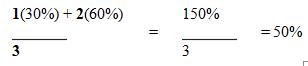Alternatively, you can calculate the actual number of silver cars and divide by the total number of cars. 40(0.3) + 80(0.6) = 12 + 48 = 60. 60/120 = 50%.

QUESTION: 3

### Paul's income is 40% less than Rex's income, Quentin's income is 20% less than Paul's income, and Sam's income is 40% less than Paul's income. If Rex gave 60% of his income to Sam and 40% of his income to Quentin, Quentin's new income would be what fraction of Sam's new income?

Solution:

Notice that Paul’s income is expressed as a percentage of Rex’s and that the other two incomes are expressed as a percent of Paul’s. Let’s assign a value of \$100 to Rex’s income. Paul’s income is 40% less than Rex's income, so (0.6)(\$100) = \$60. Quentin’s income is 20% less than Paul's income, so (0.8)(\$60) = \$48. Sam’s income is 40% less than Paul's income, so (0.6)(\$60) = \$36. If Rex gives 60% of his income, or \$60, to Sam, and 40% of his income, or \$40, to Quentin, then: Sam would have \$36 + \$60 = \$96 and Quentin would have \$48 + \$40 = \$88. Quentin’s income would now be \$88/\$96 = 11/12 that of Sam's.

QUESTION: 4

A school’s annual budget for the purchase of student computers increased by 60% this year over last year. If
the price of student computers increased by 20% this year, then the number of computers it can purchase
this year is what percent greater than the number of computers it purchased last year?

Solution:

Let’s denote the formula for the money spent on computers as pq = b, where
p = price of computers
q = quantity of computers
b = budget

We can solve a percent question that doesn’t involve actual values by using smart numbers. Let’s assign a smart number of 1000 to last year’s computer budget (b) and a smart number 100 to last year’s computer price (p).  1000 and 100 are easy numbers to take a percent of.

This year’s budget will equal 1000 × 1.6 = 1600
This year’s computer price will equal 100 × 1.2 = 120

Now we can calculate the number of computers purchased each year, q = b/p
Number of computers purchased last year = 1000/100 = 10
Number of computers purchased this year = 1600/120 = 13 1/3 (while 1/3 of a computer doesn’t make sense it won’t affect the calculation)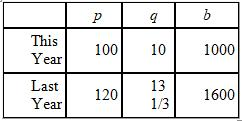The question is asking for the percent increase in quantity from last year to this year =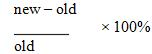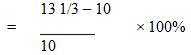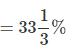This question could also have been solved algebraically by converting the percent increases into fractions.

Last year: pq = b, so q = b/p
This year: (6/5)(p)(x) = (8/5)b
If we solve for x (this year's quantity), we get x = (8/5)(5/6)b/p  or (4/3)b/p
If this year's quantity is 4/3 of last year's quantity (b/p), this represents a 33 1/3% increase.

QUESTION: 5

Boomtown urban planners expect the city’s population to increase by 10% per year over the next two years.
If that projection were to come true, the population two years from now would be exactly double the
population of one year ago. Which of the following is closest to the percent population increase in Boomtown
over the last year?

Solution:

This problem can be solved most easily with the help of smart numbers. With problems involving percentages, 100 is typically the ‘smartest’ of the smart numbers.

If we assume that today’s population is 100, next year it would be 1.1 × 100 = 110, and the following year it would be 1.1 × 110 = 121. If this is double the population of one year ago, the population at that time must have been 0.5 × 121 = 60.5. Because the problem seeks the “closest” answer choice, we can round 60.5 to 60.

In this scenario, the population has increased from 60 to 100 over the last year, a net increase of 40 residents. To determine the percentage increase over the last year, divide the net increase by the initial population: 40/60 = 4/6 = 2/3, or roughly 67%.

For those who prefer the algebraic approach: let the current population equal p. Next year the population will equal 1.1p, and the following year it will equal 1.1 × 1.1p = 1.21p. Because the question asks for the closest answer choice, we can simplify our algebra by rounding 1.21p to 1.2p. Half of 1.2p equals 0.6p. The population increase would be equal to 0.4p/0.6p = 0.4/0.6 = 2/3, or roughly 67%.

QUESTION: 6

A retailer bought a shirt at wholesale and marked it up 80% to its initial retail price of \$45. By how many
more dollars does he need to increase the price to achieve a 100% markup?

Solution:

To solve this problem, first find the wholesale price of the shirt, then compute the price required for a 100% markup, then subtract the \$45 initial retail price to get the required increase.

Let x equal the wholesale price of the shirt. The retailer marked up the wholesale price by 80% so the initial retail price is x + (80% of x). The following equation expresses the relationship mathematically:

x + 0.80x = 45
1.8x = 45
x = 45/1.8
x = 450/18
x = 25

Since the wholesale price is \$25, the price for a 100% markup is \$50. Therefore the retailer needs to increase the \$45 initial retail price by \$5 to achieve a 100% markup.

QUESTION: 7

A certain NYC taxi driver has decided to start charging a rate of r cents per person per mile. How much, in
dollars, would it cost 3 people to travel x miles if he decides to give them a 50% discount?

Solution:

We can solve this as a VIC (Variable In answer Choices) and plug in values for x and r.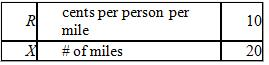Since there are 3 people, the taxi driver will charge them 30 cents per mile.
Since they want to travel 20 miles, the total charge (no discount) would be (30)(20) = 600.
With a 50% discount, the total charge will be 300 cents or 3 dollars.
If we plug r = 10 and x = 20 into the answer choices, the only answer that yields 3 dollars is D.

QUESTION: 8

Bob just filled his car's gas tank with 20 gallons of gasohol, a mixture consisting of 5% ethanol and 95%
gasoline. If his car runs best on a mixture consisting of 10% ethanol and 90% gasoline, how many gallons of
ethanol must he add into the gas tank for his car to achieve optimum performance?

Solution:

Bob put 20 gallons of gasohol into his car's gas tank, consisting of 5% ethanol and 95% gasoline. Chemical Mixture questions can be solved by using a mixture chart.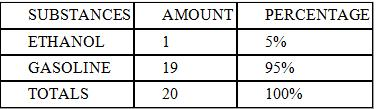This chart indicates that there is 1 gallon of ethanol out of the full 20 gallons, since 5% of 20 gallons is 1 gallon.

Now we want to add x gallons of ethanol to raise it to a 10% ethanol mixture. We can use another mixture chart to represent the altered solution.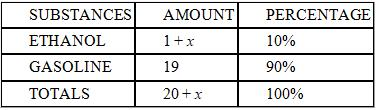Therefore, the following equation can be used to solve for x: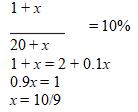QUESTION: 9

Which of the following values is closest to 1/3 + 0.4 + 65%?

Solution:

Noting that 65% is very close to 2/3, we may approximate the original expression as follows:

1/3 + 0.4 + 65%     Original expression

1/3 + 0.4 + 2/3       Close approximation

1 + 0.4

1.4

QUESTION: 10

A certain tank is filled to one quarter of its capacity with a mixture consisting of water and sodium chloride.
The proportion of sodium chloride in the tank is 40% by volume and the capacity of the tank is 24 gallons. If
the water evaporates from the tank at the rate of 0.5 gallons per hour, and the amount of sodium chloride
stays the same, what will be the concentration of water in the mixture in 2 hours?

Solution:

First, let’s find the initial amount of water in the tank:
Total mixture in the tank =1/4 × (capacity of the tank) = (1/4) × 24 = 6 gallons
Concentration of water in the mixture = 100% – (concentration of sodium chloride) = 100% – 40% = 60%
Initial amount of water in the tank = 60% × (total mixture)= 0.6 × 6 = 3.6 gallons

Next, let’s find the amount and concentration of water after 2 hours:
Amount of water that will evaporate in 2 hours = (rate of evaporation)(time) = 0.5(2) = 1 gallon
Remaining amount of water = initial amount – evaporated water = 3.6 – 1 = 2.6 gallons
Remaining amount of mixture = initial amount – evaporated water = 6 – 1 = 5 gallons
Concentration of water in the mixture in 2 hours =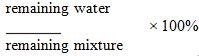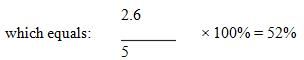QUESTION: 11

The useful life of a certain piece of equipment is determined by the following formula: u =(8d)/h2, where u is
the useful life of the equipment, in years, d is the density of the underlying material, in g/cm3, and h is the
number of hours of daily usage of the equipment. If the density of the underlying material is doubled and the
daily usage of the equipment is halved, what will be the percentage increase in the useful life of the
equipment?

Solution:

One of the most effective ways to solve problems involving formulas is to pick numbers. Note that since we are not given actual values but are asked to compute only the relative change in the useful life, we can select easy numbers and plug them into the formula to compute the percentage increase. Let’s pick d = 3 and h = 2 to simplify our computations:

Before the change: d = 3, h = 2; u = (8)(3)/22 = 24/4 = 6
After the change: d = (2)(3)= 6, h =2/2 =1; u = (8)(6)/12 = 48

Finally, percent increase is found by first calculating the change in value divided by the original value and then multiplying by 100:
(48 – 6)/6 = (42/6) = 7
(7)(100) = 700%

QUESTION: 12

If m > 0, y > 0, and x is m percent of 2y, then, in terms of y, m is what percent of x?

Solution:

Since there are variables in the answer choices, as well as in the question stem, one way to approach this problem is to pick numbers and test each answer choice. We know that x is m percent of 2y, so pick values for m and y, then solve for x.
y = 100
m = 40
x is m percent of 2y, or x is 40 percent of 200, so x = (0.40)(200) = 80.

So, for the numbers we are using, m is what percent of x? Well, m = 40, which is half of x = 80. Thus, m is 50 percent of x. The answer choice that equals 50 will be the correct choice.

(A) y/200 = 100/200 = 0.5 WRONG
(B) 2y = (2)(100) = 200 WRONG
(C) 50y = (50)(100) = 5000 WRONG
(D) 50/y = 50/100 = 0.5 WRONG
(E) 5000/y = 5000/100 = 50 CORRECT

Alternatively, we can pursue an algebraic solution.

We are given the fact that x is m percent of 2y, or x = (m/100)(2y) = my/50. Since the question asks about m (“m is what percent of x?”), we should solve this equation for m to get m = (50/y)(x).

Putting the question “m is what percent of x?” into equation form, with the word “Answer” as a placeholder, m = (Answer/100)(x).

Now we have two equations for m. If we set them equal, we can solve for the “Answer.”

QUESTION: 13

x% of y is increased by x%. What is the result in terms of x and y?

Solution:

The easiest way to solve this problem is to use the VIC method of substituting actual numbers for x and y. The problem asks us to take x% of y and increase it by x%. Since we are dealing with percentages, and the whole (y) is neither given to us nor asked of us, let's set y = 100 and x = 10. Note that this is a variation on the typical method of picking small numbers in VIC problems.

10% of 100 is 10. Increasing that 10 by 10% gives us 10 + 1 = 11. Therefore 11 is our target number. Let's test each answer choice in turn to see which of them matches our target number.

(A) 100xy + x = 100(10)(100) + 10 which doesn't equal 11.

(B) xy + x/100 = 10(100) + 10/100 which doesn't equal 11.

(C) 100xy + x/100 = 100(10)(100) + 10/100 which doesn't equal 11.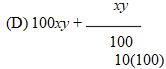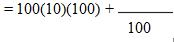which doesn't equal 11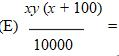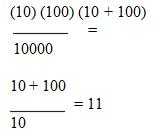QUESTION: 14

The manufacturer’s suggested retail price (MSRP) of a certain item is \$60. Store A sells the item for 20
percent more than the MSRP. The regular price of the item at Store B is 30 percent more than the MSRP, but
the item is currently on sale for 10 percent less than the regular price. If sales tax is 5 percent of the
purchase price at both stores, what is the result when the total cost of the item at Store B is subtracted from
the total cost of the item at Store A?

Solution:

First, determine the total cost of the item at each store.

Store A:
\$60 (MSRP)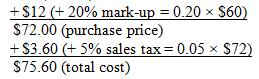Store B:
\$60 (MSRP)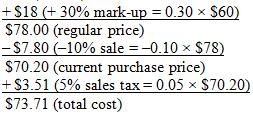The difference in total cost, subtracting the Store B cost from the Store A cost, is thus \$75.60 - \$73.71 = \$1.89.

QUESTION: 15

Two years ago, Sam put \$1,000 into a savings account. At the end of the first year, his account had accrued
\$100 in interest bringing his total balance to \$1,100. The next year, his account balance increased by 10%. At
the end of the two years, by what percent has Sam's account balance increased from his initial deposit of
\$1,000?

Solution:

Given an initial deposit of \$1,000, we must figure out the ending balance to calculate the total percent change.

After the first year, Sam's account has increased by \$100 to \$1,100.

After the second year, Sam's account again increased by 10%, but we must take 10% of \$1,100, or \$110. Thus the ending balance is \$1,210 (\$1,100 + \$110).

To calculate the percent change, we first calculate the difference between the ending balance and the initial balance: \$1,210 – \$1,000 = \$210. We divide this difference by the initial balance of \$1,000 and we get \$210/\$1,000 = .21 = 21%.